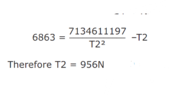# How to Derive Answers Using N Formula

• MHB
• DYLAN4321

#### DYLAN4321

Hi,

I have been given the attachment formula and asked to enter this into an excel spreadsheet. Although I am not entirely sure how the answer was derived. Is anyone able to explain step by step as I want to try and enter this into an excel spreadsheet. For reference N = Newtons

#### Attachments

•equation.png
5.7 KB · Views: 46
Hi,

I have been given the attachment formula and asked to enter this into an excel spreadsheet. Although I am not entirely sure how the answer was derived. Is anyone able to explain step by step as I want to try and enter this into an excel spreadsheet. For reference N = Newtons
I believe you were suggested to look up a solution method, the Newton-Raphson approximation being one method mentioned. Do you have a solution method you would like to use? Excel will not simply solve it for you.

-Dan

There is no "N" in the given equation so there can be no "N" in the answer! Have you left something out?

I have been given the attachment formula and asked to enter this into an excel spreadsheet.
It is not clear what your Excel formula is supposed to compute: $$\displaystyle \frac{7134611197}{T_2^2}-T_2$$ for the given value of $T_2$, the value of $T_2$ for the given left-hand side of this equation or something else. Also, for $T_2=956$ we have $$\displaystyle \frac{7134611197}{T_2^2}-T_2\approx6850$$ and not $6863$.

I believe you were suggested to look up a solution method, the Newton-Raphson approximation being one method mentioned.
Mentioned where? This is a quadratic equation in $T_2$.

There is no "N" in the given equation
N is the units in which $T_2$ is measured.

Mentioned where? This is a quadratic equation in $T_2$.
The OP also posted this on another site. Sorry, I should have included the link to it.

-Dan